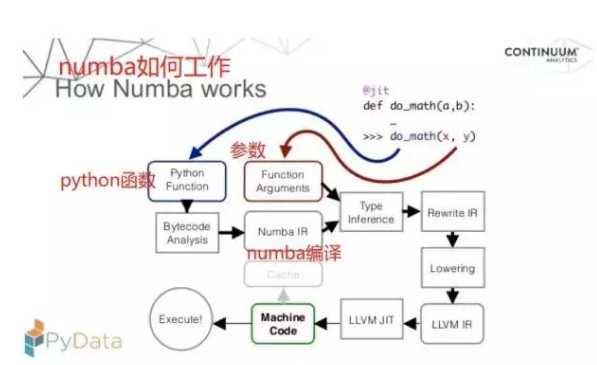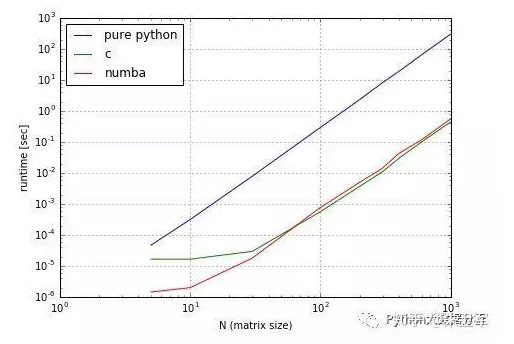# 让Python速度提升百倍的编译器——numba• 爱喝马黛茶的安东尼   2019-10-11 17:55:22570python由于它动态解释性语言的特性，跑起代码来相比java、c++要慢很多，尤其在做科学计算的时候，十亿百亿级别的运算，让python的这种劣势更加凸显。numba是一款可以将python函数编译为机器代码的JIT编译器，经过numba编译的python代码（仅限数组运算），其运行速度可以接近C或FORTRAN语言。numba工作流程

python之所以慢，是因为它是靠CPython编译的，numba的作用是给python换一种编译器。python、c、numba三种编译器速度对比

```import numba
from numba import jit
@jit(nopython=True) # jit，numba装饰器中的一种
def go_fast(a): # 首次调用时，函数被编译为机器代码
trace = 0
# 假设输入变量是numpy数组
for i in range(a.shape):   # Numba 擅长处理循环
trace += np.tanh(a[i, i])
return a + trace```

numba适合科学计算

numpy是为面向numpy数组的计算任务而设计的。

·使用numpy数组做大量科学计算时

·使用for循环时

```import numpy as np
import numba
from numba import jit```

```# 传入jit，numba装饰器中的一种
@jit(nopython=True)
def go_fast(a): # 首次调用时，函数被编译为机器代码
trace = 0
# 假设输入变量是numpy数组
for i in range(a.shape):   # Numba 擅长处理循环
trace += np.tanh(a[i, i])  # numba喜欢numpy函数
return a + trace # numba喜欢numpy广播```

nopython = True选项要求完全编译该函数（以便完全删除Python解释器调用），否则会引发异常。这些异常通常表示函数中需要修改的位置，以实现优于Python的性能。强烈建议您始终使用nopython = True。

```# 因为函数要求传入的参数是nunpy数组
x = np.arange(100).reshape(10, 10)
# 执行函数
go_fast(x)```

`% timeit go_fast(x)`

`3.63 µs ± 156 ns per loop (mean ± std. dev. of 7 runs, 100000 loops each)`

```def go_fast(a): # 首次调用时，函数被编译为机器代码
trace = 0
# 假设输入变量是numpy数组
for i in range(a.shape):   # Numba 擅长处理循环
trace += np.tanh(a[i, i])  # numba喜欢numpy函数
return a + trace # numba喜欢numpy广播
x = np.arange(100).reshape(10, 10)
%timeit go_fast(x)```

`136 µs ± 1.09 µs per loop (mean ± std. dev. of 7 runs, 10000 loops each)`

numba让python飞起来

```# 不使用numba的情况
def t():
x = 0
for i in np.arange(5000):
x += i
return x
%timeit(t())```

`408 µs ± 9.73 µs per loop (mean ± std. dev. of 7 runs, 1000 loops each)`
```# 使用numba的情况
@jit(nopython=True)
def t():
x = 0
for i in np.arange(5000):
x += i
return x
%timeit(t())```

`1.57 µs ± 53.8 ns per loop (mean ± std. dev. of 7 runs, 1000000 loops each)`

numba对python代码运行速度有巨大的提升，这极大的促进了大数据时代的python数据分析能力，对数据科学工作者来说，这真是一个lucky tool ！